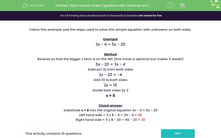# Solve Simple Linear Equations with Unknowns on Each Side

In this worksheet, students will practise solving simple linear equations where there are unknowns on each side.Key stage:  KS 3

Curriculum topic:   Algebra

Curriculum subtopic:   Solve Linear Equations (One Variable)

Popular topics:   Algebra worksheets

Difficulty level:#### Worksheet Overview

Follow this example and the steps used to solve this simple equation with unknowns on both sides.

Example

3x - 4 = 5x - 20

Method

Reverse so that the bigger x term is on the left (this move is optional but makes it easier)

5x - 20 = 3x - 4

Subtract 3x from both sides:

2x - 20 = -4

2x = 16

Divide both sides by 2:

x = 8

Substitute x = 8 into the original equation 3x - 4 = 5x - 20

Left-hand side = 3 x 8 - 4 = 24 - 4 = 20

Right-hand side = 5 x 8 - 20 = 40 - 20 = 20

Let's have a go at some questions now.

### What is EdPlace?

We're your National Curriculum aligned online education content provider helping each child succeed in English, maths and science from year 1 to GCSE. With an EdPlace account you’ll be able to track and measure progress, helping each child achieve their best. We build confidence and attainment by personalising each child’s learning at a level that suits them.

Get started